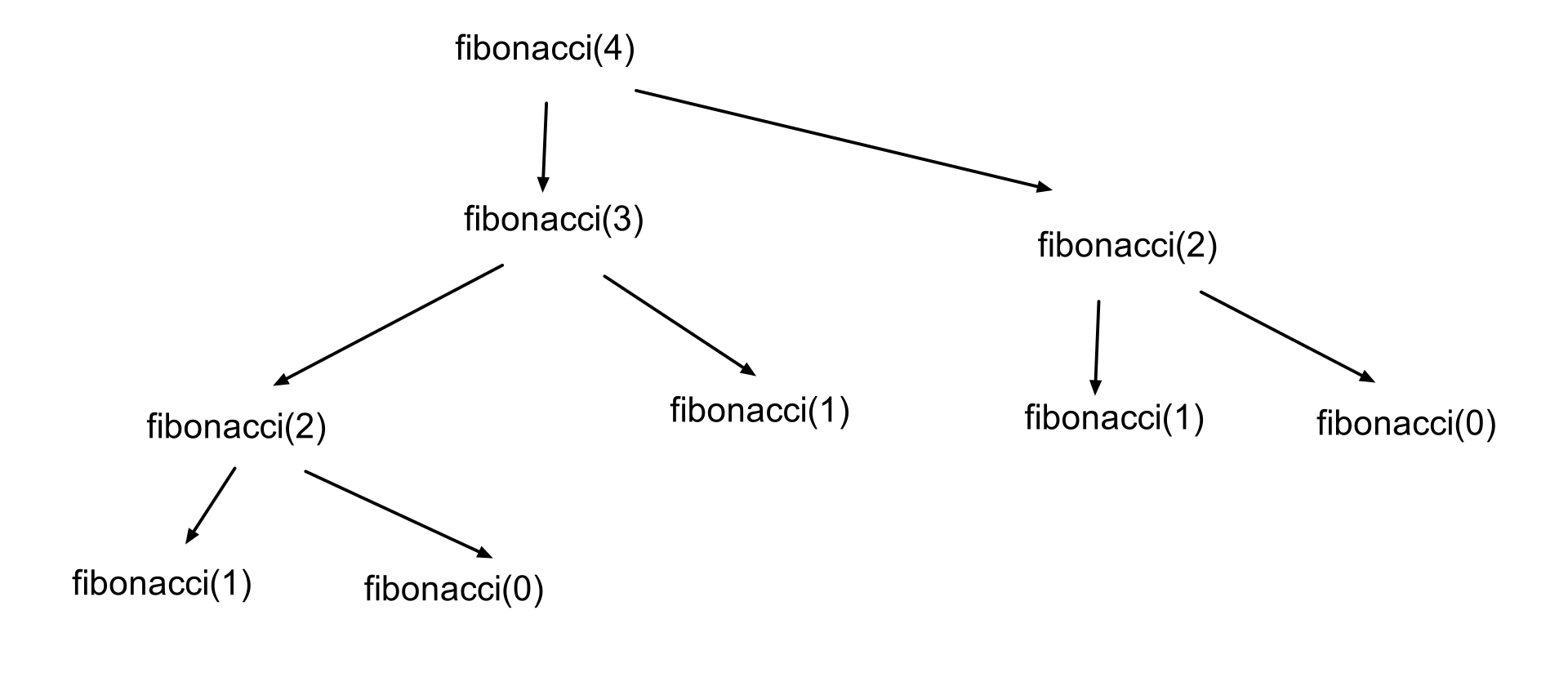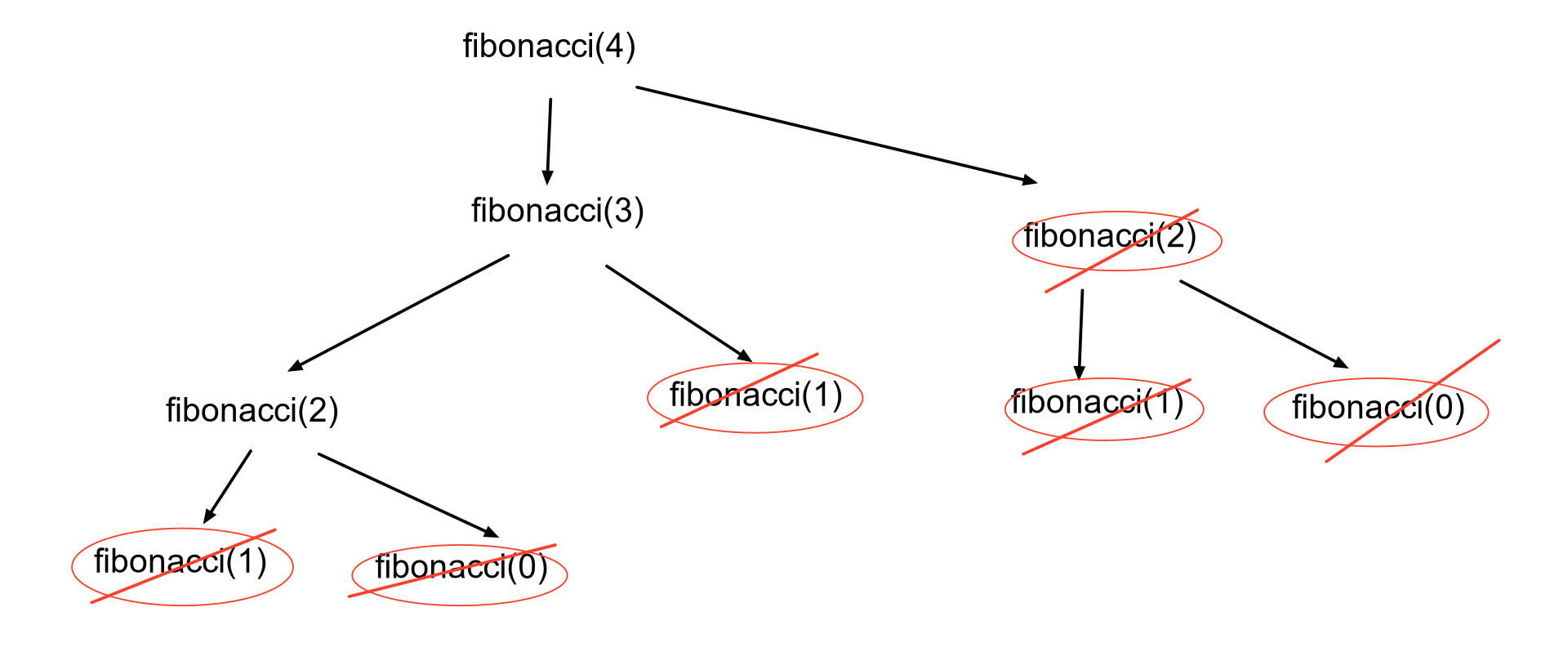# Dynamic Programming

Dynamic programming sounds very simple conceptually, but can quickly get complex.

The basic idea is “memoization” - storing previous values in memory. Under certain circumstances, you need to keep track of previous values. Dynamic programming is frequently useful as a second layer on top of recursive programming. If our recursive calls are repeating the same work, we can instead maintain a cache and eliminate the need for duplicate work.

``````def fibonacci(count):
if count == 0:
return 0
elif count == 1:
return 1
else:
return fibonacci(count - 1) + fibonacci(count - 2)
``````

This simple example will completely balloon out of control as `count` increases. Let’s look at the recursive calls for `fibonacci(4)`:Each node has two children, and then each of those children will also have two children. The runtime for this method is a whopping `O(2^n)`.

Now let’s implement fibonacci, but whenever we calculate a value, we save it to memory.

``````memo = {
0: 0,
1: 1
}
def fibonacci(count, memo):
if count in memo:
return memo[count]
else:
fib = fibonacci(count - 1, memo) + fibonacci(count - 2, memo)
memo[count] = fib
return fib
``````

This function could be less than six lines, but hopefully you will find it more understandable this way. If we look at the tree above, it will be cut down quite a bit. We will only have to calculate a value the first time it comes up.This brings our time complexity down to a handy `O(n)`, because we only have to calculate once for each value.

## Top-down or Bottom-up

If you look at the above code for fibonacci, it is a pretty classic recursive problem. Each function call breaks it down until we hit a base case. This would frequently be called top down. Conceptually, we start from the top, and work our way down the tree. However, there is another way we could think about this. Unlike normal divide-and-conquer recursive problems, we are not actually solving the entire tree. Since we know what section of the tree we are solving, we could take a bottom-up approach, and start from 0.

``````def fibonacci(count):
memo = [0, 1]
for i in range(2, count + 1):
fib = memo[i - 1] + memo[i - 2]
memo.append(fib)
return memo[count]
``````

This iterative approach is acceptable in this case. Although our original idea of fibonacci was as a tree, in the end we only truly traverse through a straight line. That allows for an iterative approach. Note that sometimes, an iterative approach is not possible.

Figuring out whether to go top-down or bottom-up, or even figuring out how to implement each of those, is the complexity of dynamic programming.

## A Harder Problem

Fibonacci is very well known. Let’s try on a different algorithm for size. Here, I will borrow a question from leetcode, written by pbrother. Here is the prompt:

``````Given an unsorted array of integers, find the length of the longest increasing
subsequence.

Input: [10,9,2,5,3,7,101,18,1]
Output: 4
Explanation: The longest increasing subsequence is [2,3,7,101], therefore the
length is 4.
``````

Note that `[2,3,7,18]` is also a valid best-subsequence.

Here I will start by taking a bottom-up approach. I will build out a list of the best subsequences that end at each index. It will look something like this:

``````opt(index) = [longest increasing subsequence UP TO THIS INDEX]
opt(0) = 
opt(1) = 
opt(2) = 
opt(3) = [2,5]
opt(4) = [2,3]
opt(5) = [2,3,7] # [2,5,7] would also be valid
opt(6) = [2,3,7,101]
opt(7) = [2,3,7,18]
opt(8) = 
``````

If I have a list of optimal subsequences for each previous index, I can easily find the optimal subsequence for the current index. I just have to find the longest subsequence that I can append the current value to.

This is the key of dynamic programming. You are building your cache to refer to while simultaneously solving the problem.

Now I will write out a solution. There are a few things to keep in mind.

1. For each index, I want to keep track of the longest subsequence at that index.
2. I will build the subsequence for the next index based on the previous ones.

In my solution, I will simplify the arrays a bit. Instead of keeping track of an entire array for each index, I will just keep track of the last value (and the length of what the array would be). Remember that the problem only cares about the length of the longest increasing subsequence, not the sequence itself.

``````def lengthOfLIS(nums):
# these will keep track of our solutions for previous indices
# much like our 'memo' did above in fibonacci
optimals = []
lengths = []

for val in nums:
max_len = 1
for i in range(len(optimals)):
opt = optimals[i]
length = lengths[i]
# we are looking for something that is both INCREASING and the LONGEST
if val > opt and length + 1 > max_len:
max_len = length + 1
lengths.append(max_len)
optimals.append(val)

# since we found the longest subsequence for each index, we can just iterate
# through our list and find the longest overall
max_len = 0
for length in lengths:
if length > max_len:
max_len = length
return max_len
``````

The solution time complexity is `O(n^2)`, because of our two nested `for` loops. The space complexity is `O(n)`, because `optimals` and `lengths` are both the same length as `nums`.

Note that this can be solved as a recursive algorithm. If you decide to do so, this will help conceptualize the difference between top-down and bottom-up dynamic algorithm design.

Categories:

Updated: